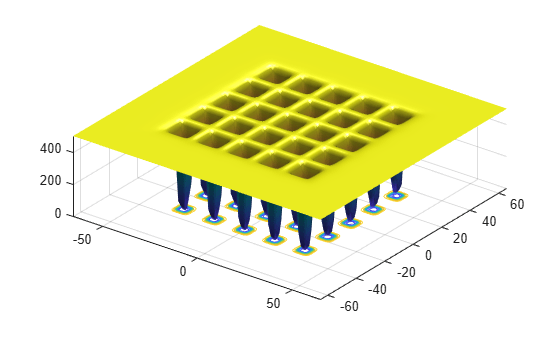# Optimize Function Using `simulannealbnd`, Problem-Based

This example shows how to minimize a function using simulated annealing in the problem-based approach when the objective is a function file, possibly of unknown content (a "black box" function). The function to minimize, `dejong5fcn(x)`, is available when you run this example. Plot the function.

`dejong5fcn`Create a 2-D optimization variable `x`. The `dejong5fcn` function expects the variable to be a row vector, so specify `x` as a 2-element row vector.

`x = optimvar("x",1,2);`

To use `dejong5fcn` as the objective function, convert the function to an optimization expression using `fcn2optimexpr`.

`fun = fcn2optimexpr(@dejong5fcn,x);`

Create an optimization problem with the objective function `fun`.

`prob = optimproblem("Objective",fun);`

Set variable bounds from –50 to 50 in all components. When you specify scalar bounds, the software expands the bounds to all variables.

```x.LowerBound = -50; x.UpperBound = 50;```

Set a pseudorandom initial point within the bounds. The initial point is a structure with field `x`.

```rng default % For reproducibility x0.x = x.LowerBound + rand(size(x.LowerBound)).*x.UpperBound;```

Solve the problem, specifying the `simulannealbnd` solver.

`[sol,fval] = solve(prob,x0,"Solver","simulannealbnd")`
```Solving problem using simulannealbnd. simulannealbnd stopped because the change in best function value is less than options.FunctionTolerance. ```
```sol = struct with fields: x: [-32.0371 -31.8792] ```
```fval = 0.9980 ```